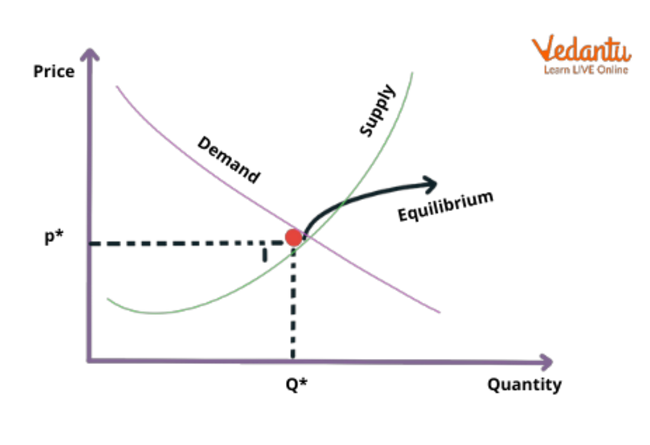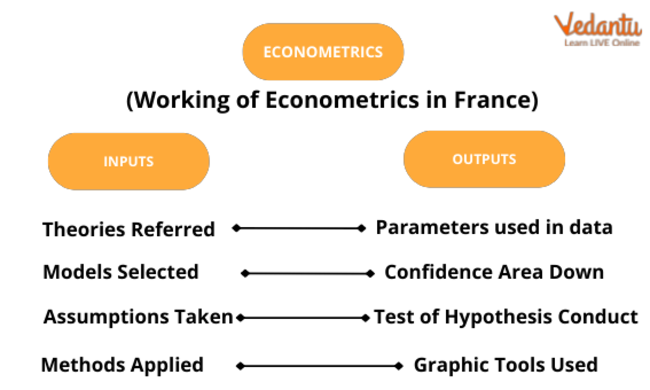Courses
Courses for Kids
Free study material
Free LIVE classes
More

# Mathematical EconomicsLIVE
Join Vedantu’s FREE Mastercalss

## What is Mathematical Economics?

Mathematical economics is a type of economic model that employs mathematical ideas and methodologies to develop economic theories and analyse economic quandaries. Maths is used by economists to do quantitative experiments and create models for predicting future economic growth.

Advances in processing power, large-data approaches, and other advanced mathematical technologies have all contributed significantly to quantitative methods being a key element of economics. All of these factors are backed by scientific approaches that promote economic research.

A new field of econometrics has emerged from the integration of statistical methodologies, mathematics, and economic concepts. The mathematical economy is a subfield of the Econometrics discipline.Use of Mathematics in Economics

## Importance of Mathematical Economics

Mathematical Economics laid the groundwork for true economic modelling. The integration of Mathematics has transformed theoretical economic models into effective tools for day-to-day economic policymaking. The purpose of econometrics, in general, is to translate qualitative assertions (such as "the association between two or more variables is positive") into quantitative statements (such as "consumption spending for every \$1 increase in discretionary income increases by 95 cents."). Mathematical Economics is extremely effective in addressing optimisation issues, such as when a policymaker seeks the optimal modification among a set of adjustments to impact a certain outcome.

## Mathematical Methods for Economics

Mathematical economics is based on mathematically specifying the important assumptions, circumstances, and causal structures of economic theories. There are two main benefits to doing this. For starters, it enables economic theorists to explain economic events and make exact inferences from their basic assumptions and definitions using mathematical techniques such as algebra and calculus.

Second, it enables economists to operationalise these theories and conclusions, allowing them to be experimentally verified using quantitative data and, if validated, utilised to provide quantitative forecasts about economic problems for the benefit of firms, investors, and politicians.

Prior to the late nineteenth century, economics depended mainly on verbal, logical reasoning, situational explanations, and anecdotal evidence-based inference to make sense of economic phenomena. Economists frequently struggled with rival models capable of describing the same recurrent relationship known as an empirical regularity, but they were unable to precisely define the extent of the correlation between important economic variables.

Mathematical economics was a departure at the time in that it presented formulae to quantify economic developments. This leaked back into economics as a whole, and most economic theories today include some form of mathematical evidence. Because of the impact of mathematical economics, decision-makers from Main Street to Wall Street to Washington have become used to hard, quantitative projections about the economy. When deciding on monetary policy, central bankers, for example, seek to know the expected impact of changes in official interest rates on inflation and the rate of economic growth. In situations like this, economists resort to econometrics and mathematical economics.

## Mathematics for Economic Analysis

Mathematical economics is the application of mathematical tools to describe ideas and evaluate economic situations. These applied approaches frequently go beyond basic geometry and may encompass differential and integral calculus, difference and differential equations, matrix algebra, mathematical programming, or other computing techniques. This strategy's proponents assert that it enables the formulation of theoretical connections with accuracy, generality, and simplicity.

• Econometrics: Mathematical economics and statistical techniques are used in econometrics in an effort to convert abstract economic ideas into practical instruments for daily economic policymaking. The overall goal of econometrics is to translate qualitative claims like "the association between two or more variables is positive" into quantitative claims like "consumption spending grows by 95 cents for every dollar increase in disposable income."

Econometrics is very effective in addressing optimisation issues, such as when a policymaker is seeking the optimum tweak out of a set of adjustments to impact a given outcome. As we become increasingly inundated with data, econometric approaches have become commonplace in economics. "Econometric approaches are utilised in many disciplines of economics, including finance, labour economics, macroeconomics, microeconomics, and economic policy," according to Stock and Watson's Introduction to Econometrics.Econometrics

Application of Mathematical Economics

Many concepts in traditional economics may be expressed using basic geometric concepts or mathematical notation. However, mathematical economics frequently uses calculus and matrix algebra in economic analysis to support strong hypotheses that would be more challenging to support otherwise. These resources are necessary for formal study in both mathematical economics and modern economic theory in general. Mathematics is the only realistic technique to approach and resolve economic problems since they frequently include so many variables. Alfred Marshall claimed that every economic issue that can be measured, expressed analytically, and resolved should be handled through mathematical work.

• Economics has become increasingly reliant on mathematical approaches, and the mathematical instruments it utilises have advanced. As a result, Mathematics has become increasingly relevant to economists and financial experts. Graduate schools in economics and finance both demand good undergraduate Mathematics preparation for admittance and, as a result, attract a rising number of mathematicians. Applied mathematicians apply mathematical concepts to practical problems such as economic analysis and other economic concerns, and many economic challenges are frequently characterised as falling within the purview of applied Mathematics.

• The framing of economic issues as stylised models with explicit assumptions and falsifiable predictions leads to this integration. This modelling might be informal and simple, as in Adam Smith's The Wealth of Nations, or formal, precise, and mathematical. Formal economic models can be classed as stochastic or deterministic, as well as discrete or continuous. In practice, quantitative modelling is used in many fields of economics, and numerous approaches have emerged independently of one another.

## Mathematical Economics Criticism

Critics warn that mathematical economics might obfuscate rather than explain the economic theory and give both theoretical and empirical economics a misleading air of accuracy and certainty. A painstakingly accurate specification of the concepts that are being regarded as quantities in a mathematical model must always be used when formulating comments regarding economic theories in mathematical terms.

Unfortunately, such a precise description is never totally achievable in economics due to the unavoidable reality that economic processes always incorporate subjective and unobservable parts that take place inside the human minds of the economic agents under consideration. This always results in interpretational difficulties and the fudge of aspects that are difficult to fit into an economic or mathematical model.

## Conclusion

The practice of mathematical economics aims to eliminate such ambiguity and fudging in order to give decision-makers and policymakers concrete, precise answers to their issues. In the best case scenario, this severely reduces the degree of assurance that can be applied to the conclusions so produced, and in the worst case scenario, complex mathematics can be utilised to mask essentially false results and conclusions.

As a result, in the interest of confidence and certitude in promoting their chosen economic explanations and policy prescriptions, economists and others who rely on them as experts and authorities tend to gloss over these concerns.

Last updated date: 29th Sep 2023
Total views: 103.8k
Views today: 2.03k

## FAQs on Mathematical Economics

1. What kind of Maths is used in Economics?

Calculus is the most commonly encountered sort of Maths in economics. Calculus is the application of several formulae to the measurement of limits, functions, and derivatives. When analysing economic data, many economists employ differential calculus. Differential calculus is the precise measurement of a derivative relating to a particular function. In its most basic form, a function represents a straight line known as a tangent. This illustrates the regular behaviour of a function. Any change in the tangent that reflects a departure (up or down) from the original line is represented by the derivative.

2. What is the role of Mathematics in Economics?

Mathematics enables economists to conduct quantified experiments and develop models for forecasting future economic growth. Advances in computer power, large-data approaches, and other sophisticated mathematical technologies have all played a significant part in making quantitative methods a key component of economics. All of these features are backed by scientific approaches that promote the study of economics. The combination of statistical methodologies, mathematics, and economic concepts has resulted in the development of an entirely new area called econometrics. The mathematical economy is a subfield of econometrics.

3. How are Maths and Economics linked?

Mathematics and economics are complementary subjects. Most aspects of contemporary economics make substantial use of mathematics and statistics, and several key areas of mathematical research have been spurred by economic concerns. Economists and mathematicians have made significant contributions to each other's fields. John Nash, a mathematician, was given the Nobel Prize in economics for his work in game theory, which has become important to modern economic theory. Haverford's Area of Concentration in Mathematical Economics allows students in both fields to not only achieve skill in the other but also to grasp the manner in which they are connected.